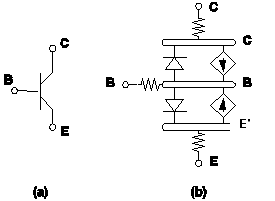Overview

The basic device elements supported by SIMPLIS -- linear resistors, linear inductors and capacitors, independent voltage and current sources, mutual inductances, four types of linear controlled sources, ideal transformers, simple switches and simple transistor switches, PWL resistors, PWL inductors and capacitors, and simple logic gates -- are very versatile and they can be used as building blocks to model a wide spectrum of electronic devices and circuits. For example, an NPN bipolar transistor can be modeled by a piecewise-linear Ebers-Moll model, by using piecewise-linear resistors for the junction diodes and two current-controlled current sources to model the current conduction. In addition, three linear resistors can be inserted to model the contact resistances as shown in 5.1

The physical transistor shown in 5.1 (a) has three nodes while the corresponding model in 5.1 (b) has six nodes, corresponding to the three terminals of the physical transistor and three internal nodes. If the physical transistor in 5.1 (a) appears only once in the entire circuit, then the model circuit in 5.1 (b) can be entered and defined in the input file as is. If the circuit contains several instances of the same device, then the model circuit in 5.1 (b) has to be repeatedly defined. For each incidence, care must be given to make sure that

1. The node names are unique compared to the other similar definitions, and
2. Unique names are given for the seven basic elements in each definition.
Obviously, this can be quite a tedious and error prone task when the size of the circuit gets larger.

If the network shown in 5.1 (b) is defined as a subcircuit instead, every incidence of the physical transistor in the circuit can be described by the following three steps:

1. give each instance of the same type of transistor a unique device name
2. define unique node names for the three terminals of each transistor
3. reference the subcircuit defining the type of transistor involved
The tedious and error prone steps involved in giving unique names to the seven device elements in the model of 5.1 (b) and giving unique names to the three internal nodes in the model are automatically handled by the subcircuit feature of SIMPLIS. The definition and the usage of the subcircuit feature of SIMPLIS is explained in detail in this chapter.5.1 Ebers-Moll model of an NPN transistor: (a) Circuit symbol, (b) the Ebers-Moll model. Note: B', C', and E' are the internal nodes introduced for modelling purposes.